## Maple 2D Output automatic aligment...

Is it possible to make executed math aka 2D Outputs, automatically align left?
I'm doing it manually for every execution I make. But is there any setting, that does orient the text to the left by standard?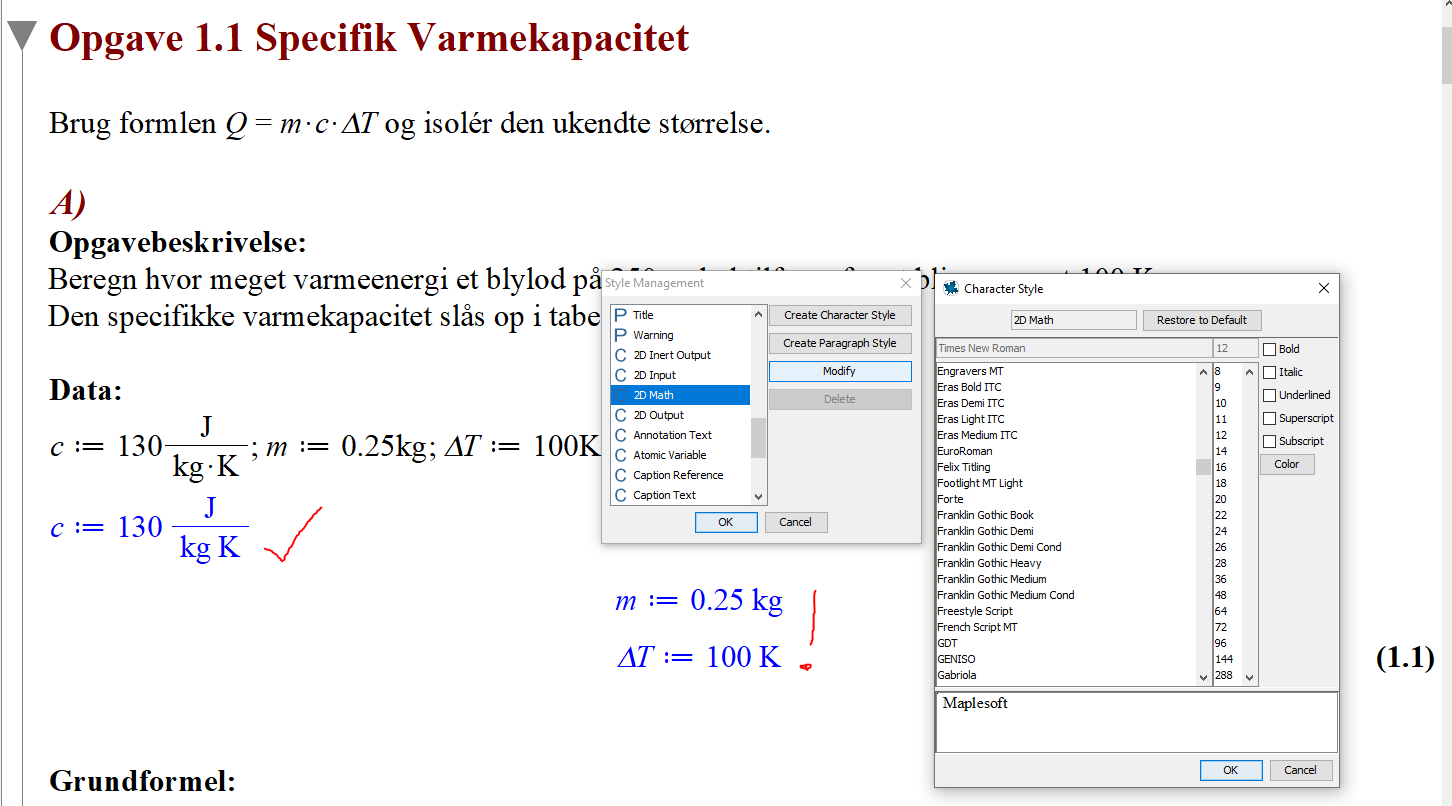## How to Perform Set Operations with Intervals?...

I have intervals A=(-infinity,7), B=(-10,25), and C=(15,infinity). How do I use Maple to perform the union and intersection of such intervals?

## new issue using Physics:-Latex() . Changes typeset...

I spend all day on this, since some tests I have were failing when I changed to using Physics:-Latex() vs. latex(). I made no change at all in my own code other than to replace latex() by Physics:-Latex().

I am still not sure why that is, but it seems due to symbol clash somewhere.  First time I run my test, it passes, next time if fails. When I change back to latex(), the test passes each time.

This seems to be due to when calling Physics:-Latex() the very first time, it automatically changing diff(y(x),x) to y'(x). May be it is storing its own x in there. (this happens on special input and not for each input, that is why only some tests fail and not all of them).

So next time I call the test it failes. May be due to different x being used. I do not know.

All what I know now is that using latex() makes my dsolve test passes each time.

But here I will show the issue which I think if fixed, should fix the main problem.

Calling Physics:-Latex() causes it to automatically change diff(y(x),x) to y'(x)  even though I am not using Typeseeting at all.

restart;
s:= y = u*x:  #special case input which causes the typesetting to kick in
ode:=diff(y(x),x)=1:
print(ode);
Physics:-Latex(s,output = string):
print(ode);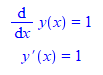Notice how the derivative changes on its own to using y'(x).  Now compare with latex()

restart;
s:= y = u*x:
ode:=diff(y(x),x)=1:
print(ode);
latex(s,output = string):
print(ode);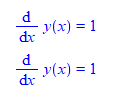So something happens inside Physics:-:Latex which causes this.

For now, I will change back to latex() until I figure more what is going on so that my test do not fail. I like to use Physics:-:Latex as it made the latex look much better.

Maple 2020.1, Physics 778

 > restart;
 > interface(version)> Physics:-Version();> s:= y = u*x: ode:=diff(y(x),x)=1: print(ode); Physics:-Latex(s,output = string): #latex(s,output = string): print(ode);>

This change of typesetting does not happen all the time. For example if s:= y = x: then it works OK. i.e. it does not change typesetting. But if s:= y = u*x: then it changes typesetting. which is very strange why that is.

I stepped into Physics:-Latex using the debugger., The typesetting changes after the call to Physics:-Latex:-Print.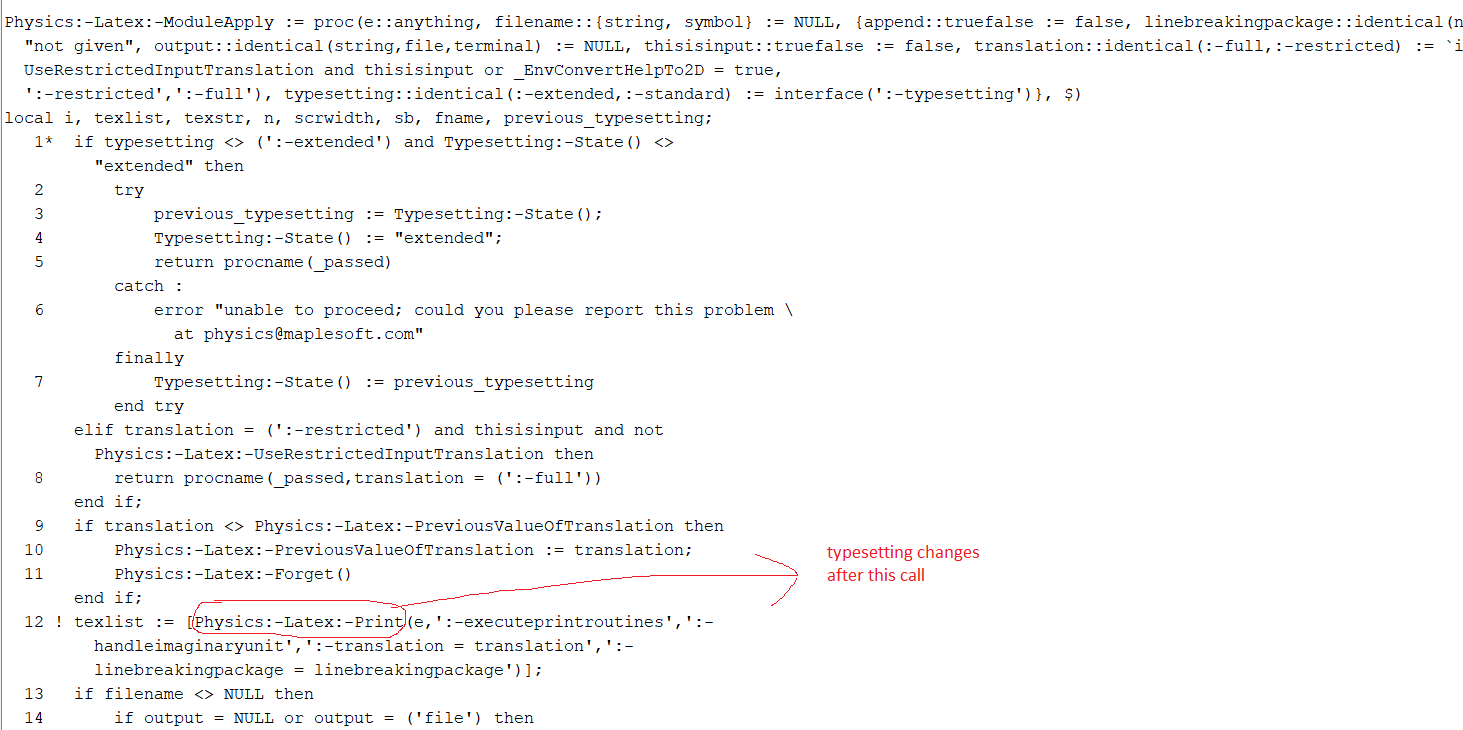## Counting transitive relations...

Determine how many transitive relations there are on a set with n elements for all positive integers n with n<=7.

## How to add a new palette...

Custom Palettes and Palette Entries on windows Pro 7
Read a tutorial from a user @les 185

https://www.mapleprimes.com/posts/207781-Custom-Palettes-And-Palette-Entries

Is this still valid this tutorial from 2015  in the current version of Maple ?
This seems to be userunfriendly.

## Trying to plot a slope field with Maple. How do ti...## Positionvector with dot notation...

There was a example in clickable calculus video showing 2D math about vectorcalculus and a positionvector fomulaes  noted with dots ( one and two dots)
How do i get this notation and is it usable in Mapleinput  1D ?

## how to generate this sequence?...

I follow below question to generate binary sequence
but number of sequence is 6 not 5, why 011001 is wrong?

number of sequence should be catalan number.

how to generate this kind of sequence correctly?

For ecah positive integer n, how many 2n-digit binary sequence
b1,b2,b2n with n "0" and n "1" are there such that
for each i=1,2,...2n, the number of "0" is larger than or equal
to the number of "1" in the subsequence b1,b2,...bi?

with(combinat):
with(ListTools):
with(SumTools):
source := permute(Flatten([seq([0,0,0,1,1,1], k=1..1)]), 6);
aavxa := []:
for z in source do
if Summation(z[k], k = 1 .. nops(z)) = 3 then
if z[nops(z)] = 1 and z = 0 then
if Reverse(z) in aavxa then
else
aavxa := [op(aavxa), z];
end if:
end if:
end if:
od:
nops(aavxa);
aavxa;

## how to make Typesetting use typesetprime=true for ...

I am learning Typesetting so I can get better Latex.

I'd like to have all derivatives generated as y'(x) and not dy/dx regardless of what the derivative variable is. (It is more clear this way).

I found I can't even give Maple a list of the variables. But must do it each time one at a time.

I have thousands of ODE's I need to typeset, (they use different variables (depending on the textbook), and not always x or t, but the set of variables is not large).

so having to check and keep changing this setting each time is awkward (but I think is doable, as I know what the independent variable is for each ode so I can call Typesetting at start of each ode processing, once I know what the variable is).

It wil be much easier if there is a way to tell Maple to do this for all variables. May be there is a good reason why Maple does not seem to allow such an option?

Here is an example

Typesetting:-Settings(usedot=false,prime=t,typesetprime=true):
ode:=diff(y(t),t$2)+diff(y(t),t)+y(t)= 0: Physics:-Latex(ode);  Which gives the Latex I want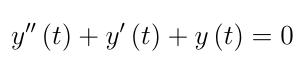But if the variable was x instead of t, I have to do this Typesetting:-Settings(usedot=false,prime=x,typesetprime=true): ode:=diff(y(x),x$2)+diff(y(x),x)+y(x)= 0:
Physics:-Latex(ode);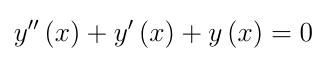I tried doing prime=[x,t] but Maple did not like this.

Is there a way or trick to tell Maple to use typesetprime=true for any letter?  I looked at https://fr.maplesoft.com/support/help/Maple/view.aspx?path=Typesetting%2fSettings but do not see a way so far.

Maple 2020.1 with Physics 776

## some issues using new Physics:-Latex() command ...

I am trying the new Physics:-Latex command in version 774.

Couple of isssues I found:

1) One test I have failed with this error

Error, (in anonymous procedure called from anonymous procedure called from anonymous procedure called from anonymous procedure called from Physics:-*) invalid boolean expression: NULL

Using the normal latex() command works OK. I hope it is not too hard to fix since this new version seems to have fixed the fraction problem in latex(). Please see

restart;
sol:=dsolve(t*(t-2)^2*diff(diff(y(t),t),t)+t*diff(y(t),t)+y(t) = 0,y(t));
latex(sol);  #OK

Physics:-Latex(sol); #error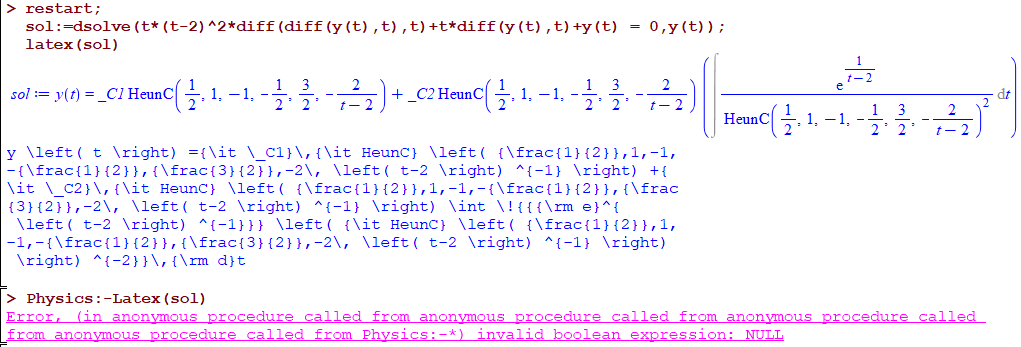2) Maple now generates Latex using maple macro, which I do not know what it is.  Comparing

restart;
ode:=t*(t-2)^2*diff(diff(y(t),t),t)+t*diff(y(t),t)+y(t) = 0;
latex(ode)


which gives

t \left( t-2 \right) ^{2}{\frac {{\rm d}^{2}}{{\rm d}{t}^{2}}}y
\left( t \right) +t{\frac {\rm d}{{\rm d}t}}y \left( t \right) +y
\left( t \right) =0


compare to

Physics:-Latex(ode)

which gives

t \left(t -2\right)^{2} \Mapleoverset{\ldots}{y}\left(t \right)
+t \Mapleoverset{.}{y}\left(t \right)+y \left(t \right) = 0


What is \Mapleoverset ? googling around, these look like part of internal Maple style sheet for Latex?

Does this mean when using the new Physics Latex command, now one needs to use the package maplestd2e.sty?  With the normal latex() command, this was not needed.

Just trying to understand the new setup.

## How do I substitute an expression to a variable in...

diff(x(t), t, t) := -R*diff(psi(t), t)*diff(phi(t), t)*sin(phi(t)) + R*diff(psi(t), t, t)*cos(phi(t)) <---- this is my expression xdoubledot

I want to substitute it in following equation

Eq__&lambda;1 := diff(x(t), t, t) - R*diff(psi(t), t, t)*cos(phi(t)) + R*diff(psi(t), t)*diff(phi(t), t)*sin(phi(t))  <----this is my equation

I have turned on physics and algebra mobdules. it still not substitiutes. even though you see diff here I have dots in my maple output I don't know why it changes when I copy paste here.

I tried subs, solve,simplify commands. None of them works

## Get a numerical value of dsolve solution and use i...

Hello,

I'm new to Maple and I'm struggling with dsolve output.

I solve this equation

ode:=diff(y(t),t)=-(4/3)*(a/eta)*(y(t)^3+1):
lambdaE:=dsolve({ode,y(0)=1},y(t),numeric,method=classical[rk4],parameters=[a,eta],output=listprocedure):
lambdaE(parameters=[theta[5,1],eta0]):

tCompute:=20*(lambda-~1)/(50/60): #vector with times I need
# lambda is a 371 lines column vector

Then I need the value of the solution at each time to compute components of a matrix

J:=Matrix(371,5):
for i from 1 to 371 do
J[i,1]:=2*(lambda[i]-1/lambda[i]^2);
J[i,2]:=4*(lambda[i]^2-2/lambda[i]-3)*(lambda[i]-1/lambda[i]^2);
J[i,3]:=6*(lambda[i]^2-2/lambda[i]-3)^2*(lambda[i]-1/lambda[i]^2);
J[i,4]:=2*(1-1/lambda[i]^3);
J[i,5]:=2*(lambdaE(tCompute[i])^2/lambda[i]-(1/lambdaE(tCompute[i])*lambda[i])); #here is where I need the value
end do;

However the output in the 5th column of J is something with y(t) and t inside

1.997498088*[t = 0.0300605520000000, y(t) = 0.999829465489350]^2 - 2.002505046/([t = 0.0300605520000000, y(t) = 0.999829465489350])

Would you have a solution to get only the numerical value ?

Manon

## How to calculating gradient as function...

In old studymaterial i could calculate  a gradient in (1,-1,2)   with obselote   package linalg

f:= (x,y,z)->exp(x*y^3*z^2);

gradf(1, -1, 2); # werkt wel
[-4 exp(-4), 12 exp(-4), -4 exp(-4)]



But i couldn't do it with the LinearAlgebra  or VectorCalculus package ?
LinearAlgebra has no gradient command, so left over Vector Calculus what is the right place

## smother curve using interpolation Package ...

This is the same example in previous post,

But here my question is how to produce smother plot of date. I tried the interpolation package but it not working..

 > restart:
 > with(LinearAlgebra): with(CurveFitting): with(Interpolation): with(plots): with(Statistics): Omega:=0.1:no:=0:Delta:=0:tau0:=1:c:=0:gamma1:=0: j:=1:k1:=0:t:=1:xr:=1:Gamma:=0.1:
 > for i from -10 to 10 do d[i]:=i: b:=sum(-gamma1*tau0+I*tau0*Delta-2*a*(k-1)*xr,k=0..1): a:=1+I*c; c1:=sqrt(conjugate(a))/tau0: c2:=0.5*((conjugate(b)/sqrt(conjugate(a)))): lambda1[i]:=2*(Gamma-I*d[i])*(t-k1)/j+(Gamma-I*d[i])^2: lambda2[i]:=t*(1-j)-k1/j+1/sqrt(2)*(Gamma-I*d[i]): lambda3[i]:=c1*(t-k1)/j+c1*(Gamma-I*d[i])+c2: J1[i]:=sqrt(Pi)/sqrt(2)*(1-erf(lambda2[i])); J1mod[i]:=(Re(J1[i]))^2+(Im(J1[i]))^2:
 > g1[i]:=0.5*sqrt(Pi)*tau0*exp(c2^2)     *exp(-conjugate(a)*((k-1)*xr)^2)/(sqrt(conjugate(a)));
 > F2[i]:=map(fnormal,evalf(sqrt(2)*Int(unapply(exp(-x^2)*erf(-sqrt(2)*c1*x+lambda3[i]),x),-lambda2[i]..5,epsilon=1e-5)));
 > J2[i]:=sum(g1[i]*J1[i]+g1[i]*F2[i],k=1..1);
 > J2mod[i]:=abs(J2[i])^2: h1[i]:=map(fnormal,evalf(exp(-d[i]^2))): f[d[i]]:=map(fnormal, evalf(h1[i]*(no*J1mod[i]+Omega^2*J2mod[i]))): #f[d[i]]:=-d[i]^2*log(exp(1))+2*log(Omega)+log(J2mod[i]): #map(simplify~, %, zero): end do:
 > with(CurveFitting): ptsN1 := CodeTools:-Usage(            [seq([d[i], evalf(f[d[i]])], i = -10 .. 10)]                          ):
 memory used=3.44KiB, alloc change=0 bytes, cpu time=0ns, real time=0ns, gc time=0ns
 >
 > P1:=plot(ptsN1, color = black, linestyle = solid, thickness = 2,      color = black, axes = boxed, labelfont = ["HELVETICA", 14],      labels = ["d", "S_M(d)"]);>
 > points := [seq(x, x = -10 .. 10, 1)]: data   := [seq(evalf(f[d[i]]), i = -10 .. 10, 1)]: f := Interpolate(points, data): plot(f,-10..10);
 >>I noticed this package is not introduced in Maple 17.. what is the alternative way to produce smother curve